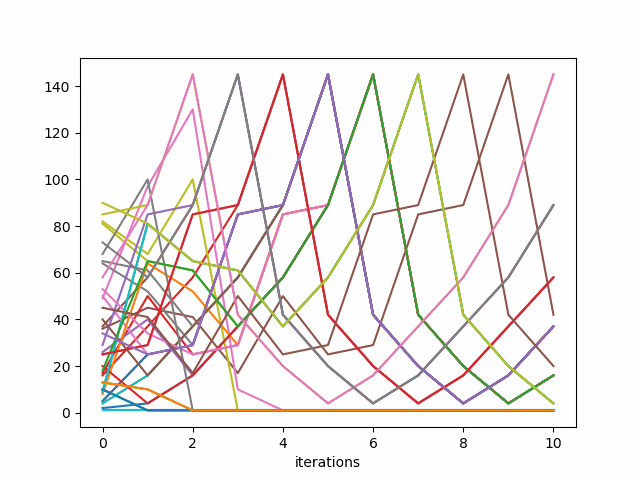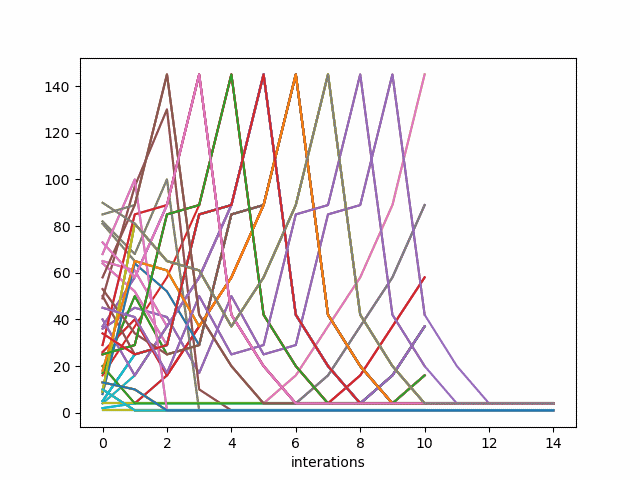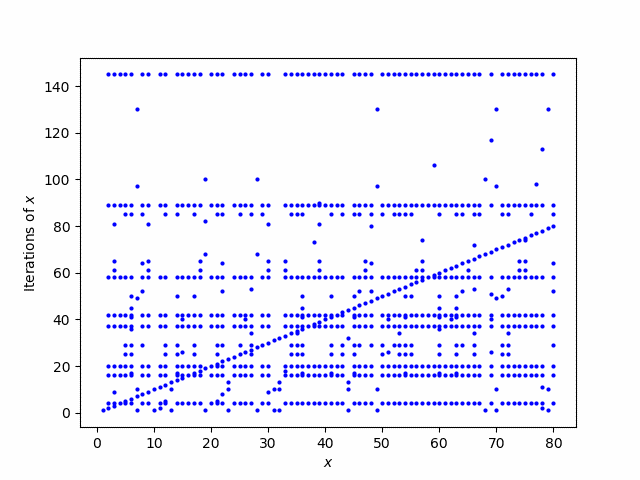# Sum of squared digits

Take a positive integer x, square each of its digits, and sum. Now do the same to the result, over and over. What happens?

To find out, let’s write a little Python code that sums the squares of the digits.

```    def G(x):
return sum(int(d)**2 for d in str(x))
```

This function turns a number into a string, and iterates over the characters in the string, turning each one back into an integer and squaring it.

Now let’s plot the trajectories of the iterations of G.

```    def iter(x, n):
for _ in range(n):
x = G(x)
return x
for x in range(1, 40):
y = [iter(x, n) for n in range(1, 12)]
plt.plot(y)
```

This produces the following plot.For every starting value, the sequence of iterations either gets stuck on 1 or it goes in the cycle 4, 16, 37, 58, 89, 145, 42, 20, 4, … . This is a theorem of A. Porges published in 1945 .

To see how the sequences eventually hit 1 or 4, let’s modify our iteration function to stop at 4 rather than cycling.

```    def iter4(x, n):
for _ in range(n):
if x != 4:
x = G(x)
return x

for x in range(1, 40):
y = [iter4(x, n) for n in range(1, 16)]
plt.plot(y)
```

This produces the following plot.Update: Here’s a better, or at least complementary, way to look at the iterations. Now the horizontal axis represents the starting points x and the points stacked vertically over x are the iterates of G starting at x.

```    def orbit(x):
pts = set()
while x not in pts:
x = G(x)
return pts

for x in range(1, 81):
for y in orbit(x):
plt.plot(x, y, "bo", markersize=2)
plt.xlabel("\$x\$")
plt.ylabel("Iterations of \$x\$")
plt.savefig("porges3.png")
```Porges, A set of eight numbers, Amer. Math. Monthly, 52(1945) 379-382.

## One thought on “Sum of squared digits”

1. Jonathan

When I was playing around with this my visualization (done with pen and paper instead of code) was a directed graph, with edges from x to G(x). I don’t think that plt has anything for this, but I’ll bet there’s a Javascript library out there that does. Time to update my skill set!

Oh, and the next fun question that arises is “What happens when you change the base?”. Since I was pen-and-papering it I never got beyond doing it in base 8 (I believe there were two different [non-trivial] cycles a number could fall into). But with code one could explore a whole range of bases.